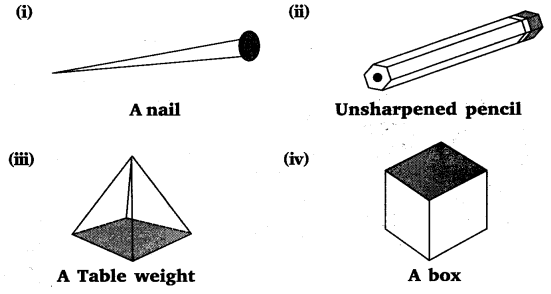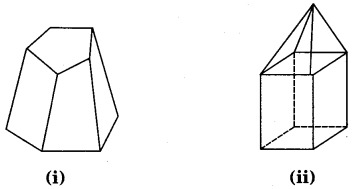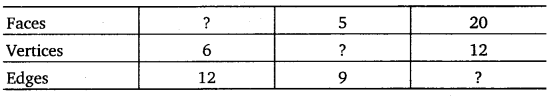# Class 8 Maths NCERT Solutions for Chapter – 10 Visualising Solid Shapes Ex – 10.3

## Visualising Solid Shapes

Question 1.
Can a polyhedron have for its faces
(i)
3 triangles?
(ii) 4 triangles?
(iii) a square and four triangles?

Solution:
Since a polyhedron is a solid, which is bounded by four or more polygonal faces in such a way that pairs of faces meet along edges and three or more edges meet in each vertex, therefore,
(i) A polyhedron cannot for its faces have three triangles.
(ii) A polyhedron can for its faces have four triangles.
(iii) A polyhedron can for its faces have a square and four triangles.

Question 2.
Is it possible to have a polyhedron with any given number of faces? (Hint : Think of a pyramid)

Solution:
Yes, it is possible only if the number of faces are greater than or equal to four.

Question 3.
Which are prisms among the following ?Solution:
We know that a prism is a polyhedron, two of whose faces are congruent polygons in parallel planes and whose other faces are parallelograms. Therefore,
(i) A nail is not a prism.
(ii) An unsharpened pencil is a prism.
(iii) A table weight is not a prism.
(iv) A box is a prism.

Question 4.
(i) How are prisms and cylinders alike?
(ii) How are pyramids and cones alike?

Solution:
(i) A prism becomes a cylinder provided the number of sides of its base becomes larger and larger.
(ii) A pyramid becomes a cone provided the number of sides of its base becomes larger and larger.

Question 5.
Is a square prism same as a cube? Explain.

Solution:
Yes, it can be a cube. But it can be a cuboid also.

Question 6.
Verify Euler’s formula for these solids.Solution:
(i) In this figure,
F = 7, V = 10, E =15
∴ F + V =7 + 10 = 17 and E + 2 =15 + 2 = 17
⇒ F+V=E+ 2
Hence, Euler’s formula is verified.

(ii) In this figure,
F =9, V = 9,E =16
∴ F + V =9 + 9 =18 and E + 2 =16 +2 =18
⇒ F + V =E + 2
Hence, Euler’s formula is verified.

Question 7.
Using Euler’s formula find the unknown.Solution:
In 1st part : Faces = ?, Vertices =6, Edges =12
By Euler’s formula, we know that F + V= E + 2
⇒ F = E + 2 – V
= 12 + 2-6 =8
Hence, faces =8

In 2nd part : Faces = 5, Vertices = ?, Edges = 9
By Euler’s formula, we know that
F + V = E + 2
⇒ V = E + 2 – F = 9+ 2- 5 = 6
Hence, vertices =6

In 3rd part : Faces =20, Vertices =12, Edges =?
By Euler’s formula, we know that
F+V=E+2
⇒ E = F + V – 2 = 20 + 12 – 2 = 30
Hence, edges =30

Question 8.
Can a polyhedron have 10 faces, 20 edges and 15 vertices?

Solution:
Since F + V ≠ E +2 (as 10 +15 ≠ 20 + 2)
A polyhedron cannot have 10 faces, 20 edges and 15 vertices.